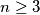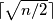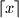### IMO Shortlist 2014 problem C5

Kvaliteta:
Avg: 0,0
Težina:
Avg: 8,0

Consider$n \geq 3$ lines in the plane such that no two lines are parallel and no three have a common point. These lines divide the plane into polygonal regions; let$\mathcal{F}$ be the set of regions having finite area. Prove that it is possible to colour$\lceil \sqrt{n / 2} \rceil$ of the lines blue in such a way that no region in$\mathcal{F}$ has a completely blue boundary. (For a real number$x$,$\lceil x \rceil$ denotes the least integer which is not smaller than$x$.)

(Austria)

Izvor: https://www.imo-official.org/problems/IMO2014SL.pdf